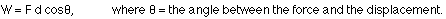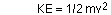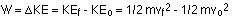## Work and energy

10-8-99

Sections 6.1 - 6.4

Energy gives us one more tool to use to analyze physical situations. When forces and accelerations are used, you usually freeze the action at a particular instant in time, draw a free-body diagram, set up force equations, figure out accelerations, etc. With energy the approach is usually a little different. Often you can look at the starting conditions (initial speed and height, for instance) and the final conditions (final speed and height), and not have to worry about what happens in between. The initial and final information can often tell you all you need to know.

### Work and energy

Whenever a force is applied to an object, causing the object to move, work is done by the force. If a force is applied but the object doesn't move, no work is done; if a force is applied and the object moves a distance d in a direction other than the direction of the force, less work is done than if the object moves a distance d in the direction of the applied force.

The physics definition of "work" is:The unit of work is the unit of energy, the joule (J). 1 J = 1 N m.

Work can be either positive or negative: if the force has a component in the same direction as the displacement of the object, the force is doing positive work. If the force has a component in the direction opposite to the displacement, the force does negative work.

If you pick a book off the floor and put it on a table, for example, you're doing positive work on the book, because you supplied an upward force and the book went up. If you pick the book up and place it gently back on the floor again, though, you're doing negative work, because the book is going down but you're exerting an upward force, acting against gravity. If you move the book at constant speed horizontally, you don't do any work on it, despite the fact that you have to exert an upward force to counter-act gravity.

### Kinetic energy

An object has kinetic energy if it has mass and if it is moving. It is energy associated with a moving object, in other words. For an object traveling at a speed v and with a mass m, the kinetic energy is given by:### The work-energy principle

There is a strong connection between work and energy, in a sense that when there is a net force doing work on an object, the object's kinetic energy will change by an amount equal to the work done:Note that the work in this equation is the work done by the net force, rather than the work done by an individual force.

### Gravitational potential energy

Let's say you're dropping a ball from a certain height, and you'd like to know how fast it's traveling the instant it hits the ground. You could apply the projectile motion equations, or you could think of the situation in terms of energy (actually, one of the projectile motion equations is really an energy equation in disguise).

If you drop an object it falls down, picking up speed along the way. This means there must be a net force on the object, doing work. This force is the force of gravity, with a magnitude equal to mg, the weight of the object. The work done by the force of gravity is the force multiplied by the distance, so if the object drops a distance h, gravity does work on the object equal to the force multiplied by the height lost, which is:

work done by gravity = W = mgh (h = height lost by the object)

An alternate way of looking at this is to call this the gravitational potential energy. An object with potential energy has the potential to do work. In the case of gravitational potential energy, the object has the potential to do work because of where it is, at a certain height above the ground, or at least above something.

### Spring potential energy

Energy can also be stored in a stretched or compressed spring. An ideal spring is one in which the amount the spring stretches or compresses is proportional to the applied force. This linear relationship between the force and the displacement is known as Hooke's law. For a spring this can be written:

F = kx, where k is known as the spring constant.

k is a measure of how difficult it is to stretch a spring. The larger k is, the stiffer the spring is and the harder the spring is to stretch.

If an object applies a force to a spring, the spring applies an equal and opposite force to the object. Therefore:

force applied by a spring : F = - kx

where x is the amount the spring is stretched. This is a restoring force, because when the spring is stretched, the force exerted by by the spring is opposite to the direction it is stretched. This accounts for the oscillating motion of a mass on a spring. If a mass hanging down from a spring is pulled down and let go, the spring exerts an upward force on the mass, moving it back to the equilibrium position, and then beyond. This compresses the spring, so the spring exerts a downward force on the mass, stopping it, and then moving it back to the equilibrium and beyond, at which point the cycle repeats. This kind of motion is known as simple harmonic motion, which we'll come back to later in the course.

The potential energy stored in a spring is given by:where x is the difference between the spring's length and its unstrained length.

In a perfect spring, no energy is lost; the energy is simply transferred back and forth between the kinetic energy of the mass on the spring and the potential energy of the spring (gravitational PE might be involved, too).

### Conservation of energy

We'll take all of the different kinds of energy we know about, and even all the other ones we don't, and relate them through one of the fundamental laws of the universe.

The law of conservation of energy states that energy can not be created or destroyed, it can merely be changed from one form of energy to another. Energy often ends up as heat, which is thermal energy (kinetic energy, really) of atoms and molecules. Kinetic friction, for example, generally turns energy into heat, and although we associate kinetic friction with energy loss, it really is just a way of transforming kinetic energy into thermal energy.

The law of conservation of energy applies always, everywhere, in any situation. There is another conservation idea associated with energy which does not apply as generally, and is therefore called a principle rather than a law. This is the principle of the conservation of mechanical energy.